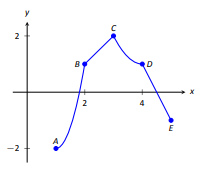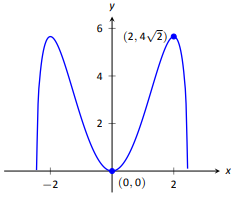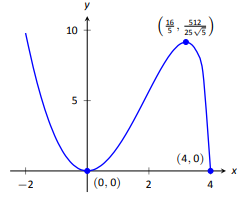$$\newcommand{\id}{\mathrm{id}}$$ $$\newcommand{\Span}{\mathrm{span}}$$ $$\newcommand{\kernel}{\mathrm{null}\,}$$ $$\newcommand{\range}{\mathrm{range}\,}$$ $$\newcommand{\RealPart}{\mathrm{Re}}$$ $$\newcommand{\ImaginaryPart}{\mathrm{Im}}$$ $$\newcommand{\Argument}{\mathrm{Arg}}$$ $$\newcommand{\norm}{\| #1 \|}$$ $$\newcommand{\inner}{\langle #1, #2 \rangle}$$ $$\newcommand{\Span}{\mathrm{span}}$$

# 3.E: Applications of the Graphical Behavior of Functions(Exercises)

$$\newcommand{\vecs}{\overset { \rightharpoonup} {\mathbf{#1}} }$$ $$\newcommand{\vecd}{\overset{-\!-\!\rightharpoonup}{\vphantom{a}\smash {#1}}}$$$$\newcommand{\id}{\mathrm{id}}$$ $$\newcommand{\Span}{\mathrm{span}}$$ $$\newcommand{\kernel}{\mathrm{null}\,}$$ $$\newcommand{\range}{\mathrm{range}\,}$$ $$\newcommand{\RealPart}{\mathrm{Re}}$$ $$\newcommand{\ImaginaryPart}{\mathrm{Im}}$$ $$\newcommand{\Argument}{\mathrm{Arg}}$$ $$\newcommand{\norm}{\| #1 \|}$$ $$\newcommand{\inner}{\langle #1, #2 \rangle}$$ $$\newcommand{\Span}{\mathrm{span}}$$ $$\newcommand{\id}{\mathrm{id}}$$ $$\newcommand{\Span}{\mathrm{span}}$$ $$\newcommand{\kernel}{\mathrm{null}\,}$$ $$\newcommand{\range}{\mathrm{range}\,}$$ $$\newcommand{\RealPart}{\mathrm{Re}}$$ $$\newcommand{\ImaginaryPart}{\mathrm{Im}}$$ $$\newcommand{\Argument}{\mathrm{Arg}}$$ $$\newcommand{\norm}{\| #1 \|}$$ $$\newcommand{\inner}{\langle #1, #2 \rangle}$$ $$\newcommand{\Span}{\mathrm{span}}$$

## 3.1: Extreme Values

Terms and Concepts

1. Describe what an "extreme value" of a function is in your own words.

2. Sketch the graph of a function $$f$$ on (-1,1) that has both a maximum and minimum value.

3. Describe the difference between absolute and relative maxima in your own words.

4. Sketch the graph of a function $$f$$ where $$f$$ has a relative maximum at $$x=1$$ and $$f'(1)$$ is undefined.

5. T/F: If $$c$$ is a critical value of a function $$f$$, then $$f$$ has either a relative maximum or relative minimum at $$x=c$$.

### Problems

In Exercises 6-7, identify each of the marked points as being an absolute maximum and minimum, a relative maximum or minimum, or none of the above.

6.7.In Exercises 8-14, evaluate $$f'(x)$$ at the points indicated in the graph.

8. $$f(x)=\frac{2}{x^2+1}$$9. $$f(x) = x^2\sqrt{6-x^2}$$10. $$f(x)=\sin x$$11. $$f(x) = x^2\sqrt{4-x}$$12. $$f(x) =\begin{cases} x^2 \quad &x\le 0 \\ x^5 &x>0 \end{cases}$$13. $$f(x) =\begin{cases} x^2 \quad &x\le 0 \\ x &x>0 \end{cases}$$14. $$f(x) = \frac{(x-2)^{2/3}}{x}$$In Exercises 15-24, find the extreme values of the function on the given interval.

15. $$f(x) =x^2+x+4\text{ on }[-1,2]$$.

16. $$f(x) =x^3-\frac{9}{2}x^2-30x+3\text{ on }[0,6]$$.

17. $$f(x) =3\sin x\text{ on }[\pi/4,2\pi/3]$$.

18. $$f(x) =x^2\sqrt{4-x^2}\text{ on }[-2,2]$$.

19. $$f(x) =x+\frac{3}{x}\text{ on }[1,5]$$.

20. $$f(x) =\frac{x^2}{x^2+5}\text{ on }[-3,5]$$.

21. $$f(x) =e^x\cos x\text{ on }[0,\pi]$$.

22. $$f(x) =e^x\sin x\text{ on }[0,\pi]$$.

23. $$f(x) =\frac{\ln x}{x}\text{ on }[1,4]$$.

24. $$f(x) =x^{2/3}-x\text{ on }[0,2]$$.

### Review

25. Find $$\frac{dy}{dx}$$, where $$x^2y-y^2x=1$$.

26. Find the equation of the line tangent to the graph of $$x^2+y^2+xy=7$$ at the point $$(1,2)$$.

27. Let $$f(x)=x^3+x$$. Evaluate $$\lim\limits_{s\to 0} \frac{f(x+s)-f(x)}{s}$$.

## 3.2: The Mean Value Theorem

Terms and Concepts

1. Explain in your own words what the Mean Value Theorem states.

2. Explain in your own words what Rolle's Theorem states.

### Problems

In Exercises 3-10, a function $$f(x)$$ and interval [a,b] are given. Check if Rolle's Theorem can be applied to $$f$$ on [a,b]; if so, find $$c$$ in [a,b] such that $$f'(c)=0$$.

3. $$f(x) =6\text{ on }[-1,1]$$.

4. $$f(x) =6x\text{ on }[-1,1]$$.

5. $$f(x) =x^2+x-6\text{ on }[-3,2]$$.

6. $$f(x) =x^2+x-2\text{ on }[-3,2]$$.

7. $$f(x) =x^2+x\text{ on }[-2,2]$$.

8. $$f(x) =\sin x \text{ on }[\pi/6,5\pi/6]$$.

9. $$f(x) =\cos x\text{ on }[0,\pi]$$.

10. $$f(x) =\frac{1}{x^2-2x+1}\text{ on }[0,2]$$.

In Exercises 11-20, a function $$f(x)$$ and interval [a,b] are given. Check if the Mean Value Theorem can be applied to $$f$$ on [a,b]; if so, find a value $$c$$ in [a,b] guaranteed by the Mean Value Theorem.

11. $$f(x) =x^2+3x-1\text{ on }[-2,2]$$.

12. $$f(x) =5x^2-6x+8\text{ on }[0,5]$$.

13. $$f(x) =\sqrt{9-x^2}\text{ on }[0,3]$$.

14. $$f(x) =\sqrt{25-x}\text{ on }[0,9]$$.

16. $$f(x) =\ln x\text{ on }[1,5]$$.

17. $$f(x) =\tan x\text{ on }[\pi/4, \pi/4]$$.

18. $$f(x) =x^3-2x^2+x+1\text{ on }[-2,2]$$.

19. $$f(x) =2x^3-5x^2+6x+1\text{ on }[-5,2]$$.

20. $$f(x) =\sin^{-1}x\text{ on }[-1,1]$$.

### Review

21. Find the extreme values of $$f(x)=x^2-3x+9\text{ on }[-2,5]$$.

22. Describe the critical points of $$f(x) =\cos x$$.

23. Describe the critical points of $$f(x)=\tan x$$.

## 3.3: Increasing and Decreasing Functions

### Terms and Concepts

1. In your own words describe what it means for a function to be increasing.

2. What does a decreasing function “look like”?

3. Sketch a graph of a function on [0,2] that is increasing but not strictly increasing.

4. Give an example of a function describing a situation where it is “bad” to be increasing and “good” to be decreasing.

5. A function f has derivative $$f ′ (x) = (\sin x + 2)e^{x^2+1}$$, where $$f ′ (x) > 1$$ for all $$x$$. Is $$f$$ increasing, decreasing, or can we not tell from the given information?

### Problems

In Exercises 6-13, a function $$f(x)$$ is given.
(a) Compute $$f'(x)$$.
(b) Graph $$f$$ and $$f'$$ on the same axes (using technology is permitted) and verify Theorem 29.

6. $$f(x) =3x+4$$

7. $$f(x) =x^2-3x+5$$

8. $$f(x) =\cos x$$

9. $$f(x) =\tan x$$

10. $$f(x) =x^3-5x^2+7x-1$$

11. $$f(x) =2x^3-x^2+x-1$$

12. $$f(x) =x^4-5x^2+4$$

13. $$f(x) =\frac{1}{x^2+1}$$

In Exercises 14-23, a function $$f(x)$$ is given.
(a) Give the domain of $$f$$.
(b) Find the critical numbers of $$f$$.
(c) Create a number line to determine the intervals on which $$f$$ is increasing and decreasing.
(d) Use the First Derivative Test to determine whether each critical point is a relative maximum, minimum, or neither.

14. $$f(x) =x^2+2x-3$$

15. $$f(x) =x^3+3x^2+3$$

16. $$f(x) =2x^3+x^2+3$$

17. $$f(x) =x^3-3x^2+3x-1$$

18. $$f(x) =\frac{1}{x^2-2x+2}$$

19. $$f(x) =\frac{x^2-4}{x^2-1}$$

20. $$f(x) =\frac{x}{x^2-2x-8}$$

21. $$f(x) =\frac{(x-2)^{2/3}}{x}$$

22. $$f(x) =\sin x\cos x\text{ on }(-\pi,\pi)$$

23. $$f(x) = 5x^2-5x$$

### Review

24. Consider $$f(x)=x^2-3x+5$$ on [-1,2]; find $$c$$ guaranteed by the Mean Value Theorem.

25. Consider $$f(x)=\sin x\text{ on }[-\pi/2, \pi/2]$$; find $$c) guaranteed by the Mean Value Theorem. ## 3.4: Concavity and the Second Derivative ### Terms and Concepts 1. Sketch a graph of a function \(f(x)$$ that is concave up on (0,1) and is concave down on (1,2).

2. Sketch a graph of a function $$f(x)$$ that is:
(a) Increasing, concave up on (0,1),
(b) increasing, concave down on (1,2),
(c) decreasing, concave down on (2,3) and
(d) increasing, concave down on (3,4).

3. Is it possible for a function to be increasing and concave down on $$(0,\infty)$$ with a horizontal asymptote of $$y=1$$? If so, give a sketch of such a function.

4. Is it possible for a function to be increasing and concave upon $$(0,\infty)$$ with a horizontal asymptote of $$y=1$$? If so, give a sketch of such a function.

### Problems

In Exercises 5-15, a function $$f(x)$$ is given.
(a) Compute $$f''(x)$$.
(b) Graph $$f \text{ and }f''$$ on the same axes (using technology is permitted) and verify Theorem 31.

5. $$f(x)=-7x+3$$

6. $$f(x)=-4x^2+3x-8$$

7. $$f(x)=4x^2+3x-8$$

8. $$f(x)=x^3-3x^2+x-1$$

9. $$f(x)=-x^3+x^2-2x+5$$

10. $$f(x)=\cos x$$

11. $$f(x)=\sin x$$

12. $$f(x) =\tan x$$

13. $$f(x)=\frac{1}{x^2+1}$$

14. $$f(x) =\frac{1}{x}$$

15. $$f(x) = \frac{1}{x^2}$$

In Exercises 16-28, a function $$f(x)$$ is given.
(a) Find possible points of inflection of $$f$$
(b) Create a number line to determine the intervals on which $$f$$ is concave up or concave down.

16. $$f(x)=x^2-2x+1$$

17. $$f(x)=-x^2-5x+7$$

18. $$f(x)=x^3-x+1$$

19. $$f(x)=2x^3-3x^2+9x+5$$

20. $$f(x)=\frac{x^4}{4}+\frac{x^3}{3}-2x+3$$

21. $$f(x)=-3x^4+8x^3+6x^2-24x+2$$

22. $$f(x)=x^4-4x^3+6x^2-4x+1$$

23. $$f(x)=\frac{1}{x^2+1}$$

24. $$f(x)=\frac{x}{x^2-1}$$

25. $$f(x)=\sin x+\cos x\text{ on }(-\pi,\pi)$$

26. $$f(x)=x^2e^x$$

27. $$f(x)=x^2\ln x$$

28. $$f(x)=e^{-x^2}$$

In Exercises 29-41, a function $$f(x)$$ is given. Find the critical points of $$f$$ and use the Second Derivative Test, when possible, to determine the relative extrema. (Note: these are the same functions as in exercises 16-28.)

29. $$f(x)=x^2-2x+1$$

30. $$f(x)=-x^2-5x+7$$

31. $$f(x)=x^3-x+1$$

32. $$f(x)=2x^3-3x^2+9x+5$$

33. $$f(x)=\frac{x^4}{4}+\frac{x^3}{3}-2x+3$$

34. $$f(x)=-3x^4+8x^3+6x^2-24x+2$$

35. $$f(x)=x^4-4x^3+6x^2-4x+1$$

36. $$f(x)=\frac{1}{x^2+1}$$

37. $$f(x)=\frac{x}{x^2-1}$$

38. $$f(x)=\sin x+\cos x\text{ on }(-\pi,\pi)$$

39. $$f(x)=x^2e^x$$

40. $$f(x)=x^2\ln x$$

41. $$f(x)=e^{-x^2}$$

In Exercises 42-54, a function $$f(x)$$ is given. Find the x values where $$f'(x)$$ has a relative maximum or minimum. (Note: these are the same functions as in Exercises 16-28.)

42. $$f(x)=x^2-2x+1$$

43. $$f(x)=-x^2-5x+7$$

44. $$f(x)=x^3-x+1$$

45. $$f(x)=2x^3-3x^2+9x+5$$

46. $$f(x)=\frac{x^4}{4}+\frac{x^3}{3}-2x+3$$

47. $$f(x)=-3x^4+8x^3+6x^2-24x+2$$

48. $$f(x)=x^4-4x^3+6x^2-4x+1$$

49. $$f(x)=\frac{1}{x^2+1}$$

50. $$f(x)=\frac{x}{x^2-1}$$

51. $$f(x)=\sin x+\cos x\text{ on }(-\pi,\pi)$$

52. $$f(x)=x^2e^x$$

53. $$f(x)=x^2\ln x$$

54. $$f(x)=e^{-x^2}$$

## 3.5: Curve Sketching

### Terms and Concepts

1. Why is sketching curves by hand beneficial even though technology is ubiquitous?

2. What does “ubiquitous” mean?

3. T/F: When sketching graphs of functions, it is useful to find the critical points.

4. T/F: When sketching graphs of functions, it is useful to find the possible points of inflection.

5. T/F: When sketching graphs of functions, it is useful to find the horizontal and vertical asymptotes.

### Problems

In Exercises 6-11, practice using Key Idea 4 by applying the principles to the given functions with familiar graphs.

6. $$f(x) =2x+4$$

7. $$f(x) =-x^2+1$$

8. $$f(x) =\sin x$$

9. $$f(x) =e^x$$

10. $$f(x) =\frac{1}{x}$$

11. $$f(x) =\frac{1}{x^2}$$

In Exercises 12-25, sketch a graph of the given function using Key Idea 4. Show all work; check your answer with technology.

12. $$f(x) =x^3-2x^2+4x+1$$

13. $$f(x) =-x^3+5x^2-3x+2$$

14. $$f(x) =x^3+3x^2+3x+1$$

15. $$f(x) =x^3-x^2-x+1$$

16. $$f(x) =(x-2)\ln (x-2)$$

17. $$f(x) =(x-2)^2\ln (x-2)$$

18. $$f(x) =\frac{x^2-4}{x^2}$$

19. $$f(x) =\frac{x^2-4x+3}{x^2-6x+8}$$

20. $$f(x) =\frac{x^2-2x+1}{x^2-6x+8}$$

21. $$f(x) =x\sqrt{x+1}$$

22. $$f(x) =x^2e^x$$

23. $$f(x) =\sin x\cos x \text{ on }[-\pi,\pi]$$

24. $$f(x) =(x-3)^{2/3}+2$$

25. $$f(x) =\frac{(x-1)^{2/3}}{x}$$

In Exercises 26-28, a function with the parameters $$a$$ and $$b$$ are given. Describe the critical points and possible points of inflection of $$f$$ in terms of $$a$$ and $$b$$.

26. $$f(x) =\frac{a}{x^2+b^2}$$

27. $$f(x) =\sin (ax+b)$$

28. $$f(x) = (x-a)(x-b)$$

29. Given $$x^2+y^2=1$$, use implicit differentiation to find $$frac{dy}{dx}$$ and $$\frac{d^2y}{dx^2}$$. Use this information to justify the sketch of the unit circle.

3.E: Applications of the Graphical Behavior of Functions(Exercises) is shared under a CC BY-NC license and was authored, remixed, and/or curated by LibreTexts.